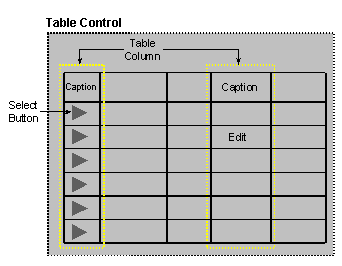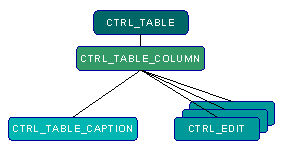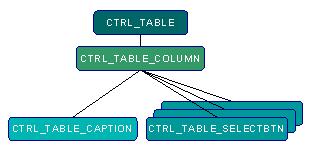Handling a Table ControlA table control is a control that contains an R/3 table data. It has columns and rows of data.

The table control is described in the the control structure (IT_CTRL). More specific information on the table control is in the IT_TABLEINFO structure.

The Controls in a Table Control

The following diagram shows the parts of a table control.Controls Hierarchy of a Table Control

The Table control ( control type CTRL_TABLE) is the parent of all the controls in the table.

The Table control is the parent control for all the Table Column controls (CTRL_TABLE_COLUMN).

Each Table Column control is the parent of the Column Caption control (CTRL_TABLE_CAPTION), which is the title of the column. In addition, the Table Column control is the parent of all the cells of data in the column, which are of CTRL_EDIT type.

The following diagram summarizes this relationship.When the table contains selection buttons (which allow you to select a specific row in the table), the column of the selection buttons also constitutes a Table Column control. In this case the Table Column control is the parent of a caption (CTRL_TABLE_CAPTION), which has no text, and it is also the parent of all the row selection buttons (CTRL_TABLE_SELECTBTN).

The following diagram summarizes this relationship.The information on the various controls which are a part of the table is in the control structure (IT_CTRL).

Using Table Controls in SAPGUI

The following operations are allowed on a table control in SAPGUI:

• Scrolling up and down to view different rows in the table
• Scrolling left and right to view more columns in the table
• A column may be defined as fixed, and then the end user cannot scroll over this column.
• Switching the location of two columns in the table, using drag and drop
• Changing the width of a column

Using Table Controls in the GUI Library

The GUI Library provides all the above operations on a table control.

Displaying a Table Control

You display a table control as you would any other control on the screen.

Working with a Table Control

The following steps describe a typical procedure for working with the table control:

1. Use ItEv_GetControlInfo, with the table control as a parameter, to get the extended information on the table control, such as the number of rows and column in it, or the number of fixed columns it contains. This fills the It_TableInfo structure with the extended table control information.
2. Change the information in the It_TableInfo structure, if needed. For example, the TabVerScrollbarStartRow determines the first row that is displayed on the screen. To scroll to a particular row, for example, change the value of this parameter to be the number of the row to be displayed as the first row after scrolling.
3. Use ItEv_SetControlInfo to apply the changes to the It_TableInfo structure.
4. Send the event to the server with It_SendEvent.
5. This will send the changes in the It_TableInfo structure to the application server.

6. Use It_GetEvent if you wish to refresh the screen with new table data.

Example: Scrolling

The following example scrolls in a table control to display row number 5 as the first row. It assumes that control number 16 on the screen is a table.

pEvt->eventtype |= MES_VSCROLL ;

IT_TABLEINFO tbinfo;
int len = ItEv_GetControlInfo(pEvt, ITCTRL_IDX(16),&tbinfo, sizeof(tbinfo));
tbinfo.TabVerScrollbarStartRow = 5;
len = ItEv_SetControlInfo(pEvt, ITCTRL_IDX(16),&tbinfo, sizeof(tbinfo));
if (It_SendEvent(hMr, &pEvt) == FALSE) {
printf("error in sendevent\n");
}

if (It_GetEvent(hMr, &pEvt) == FALSE) {

printf("error in getevent\n");

}

Switching Between Columns

Use the ItEv_SetTableColumnPermutation function to switch between two columns in the table control.

The ItEv_SetTableColumnPermutation function uses an array that stores the order of the columns in the table control. Changing the values in the array changes the order of the columns in the table control.

The following example prompts the user to enter the columns to switch, and then switches between them. It assumes that control number 16 on the screen is a table.

printf("Swap: ");
int a, b, pCols;
for (i = 0; i < 10; i++)
pCols[i] = i;
scanf("%d %d", &a, &b);
pCols[a] = b;
pCols[b] = a;
ItEv_SetTableColumnPermutation(pEvt, ITCTRL_IDX(16), pCols, 10);
It_SendEvent(hMr, &pEvt);
It_GetEvent(hMr, &pEvt);

Changing the Width of a Column

Use the ItEv_SetWidth function to change the width of a table control column.